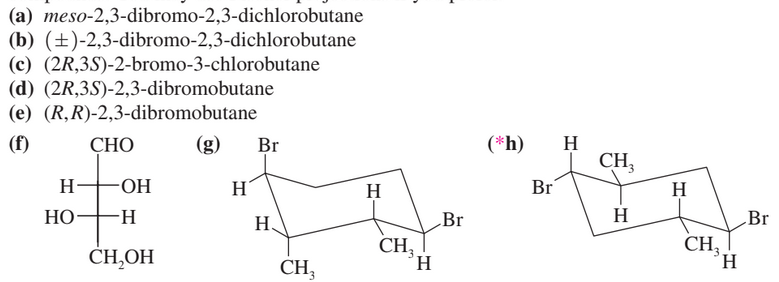×
Get Full Access to Organic Chemistry - 8 Edition - Chapter 5 - Problem 21p
Get Full Access to Organic Chemistry - 8 Edition - Chapter 5 - Problem 21p

×

# Which of the following compounds are chiral? Draw eachISBN: 9780321768414 33

## Solution for problem 21P Chapter 5

Organic Chemistry | 8th Edition

• Textbook Solutions
• 2901 Step-by-step solutions solved by professors and subject experts
• Get 24/7 help from StudySoup virtual teaching assistantsOrganic Chemistry | 8th Edition

4 5 1 272 Reviews
24
4
Problem 21P

Which of the following compounds are chiral? Draw each compound in its most symmetric conformation, star any asymmetric carbon atoms, and draw any mirror planes. Label any meso

compounds. You may use Fischer projections if you prefer.Step-by-Step Solution:

Solution 21P

Step 1Step 2 of 8

Step 3 of 8

##### ISBN: 9780321768414

Organic Chemistry was written by and is associated to the ISBN: 9780321768414. The answer to “?Which of the following compounds are chiral? Draw each compound in its most symmetric conformation, star any asymmetric carbon atoms, and draw any mirror planes. Label any meso compounds. You may use Fischer projections if you prefer.” is broken down into a number of easy to follow steps, and 37 words. This full solution covers the following key subjects: any, dichlorobutane, draw, dibromobutane, dibromo. This expansive textbook survival guide covers 25 chapters, and 1336 solutions. The full step-by-step solution to problem: 21P from chapter: 5 was answered by , our top Chemistry solution expert on 05/06/17, 06:41PM. Since the solution to 21P from 5 chapter was answered, more than 1280 students have viewed the full step-by-step answer. This textbook survival guide was created for the textbook: Organic Chemistry, edition: 8.

## Discover and learn what students are asking

Calculus: Early Transcendental Functions : Basic Differentiation Rules and Rates of Change
?Finding a Derivative In Exercises 3–24, use the rules of differentiation to find the derivative of the function. $$y=12$$

Calculus: Early Transcendental Functions : Antiderivatives and Indefinite Integration
?In Exercises 7–10, complete the table to find the indefinite integral. Original Integral Rewrite

Unlock Textbook Solution

Enter your email below to unlock your verified solution to:

Which of the following compounds are chiral? Draw each